# Working with Symbolic Expressions

## From Math for Programmers by Paul Orland

● Modeling algebraic expressions as data structures in Python

● Writing code to analyze, transform, or evaluate an algebraic expression

● Building a data structure from elements and combinators

____________________________________________________________

Take 40% off Math for Programmers. Just enter fccorland into the discount code box at checkout at manning.com.
____________________________________________________________

In Python and other languages, we often think of functions as mini-programs. They’re self-contained sets of instructions that accept some input data, do some ordered computations with it, and identify some result value as an output. From the perspective of functional programming, we consider functions to be data that we can compute things about. You’ve now seen a number of functions which take other functions as input, or produce functions as output.

How do you compute facts about functions? Say we have a mathematical function like

We can easily translate it to Python:

`from math import sin def f(x):     return (3*x**2 + x) * sin(x)`

Can we write a program to find out whether the result of f(x) depends on x, as opposed to g(x) = 7which doesn’t? Can we determine whether it contains the trigonometric sine function? Another thing we could ask is whether the expression can be expanded according to the rules of algebra. In this case it can: f(x) could be equivalently written as 3x2 sin(x) + x sin(x). If we could write a function to expand an algebraic expression, we could picture it like this: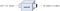Figure 1: Can we write an “expand” function that expands a mathematical expression according to the rules of algebra?

These hypothetical programs would need to inspect the body of a function, rather than just evaluating it. In this article, I’ll show you how to do this using a broadly applicable approach called symbolic programming. The key is modeling algebraic expressions as data structures, rather than translating them directly to Python code, and then they’re more amenable to manipulation.

In the book, Math for Programmers, you’ll see how to extend what we cover in this chapter to automate processes from calculus. Taking the derivative of a function, for instance, requires inspecting the form of an input function and applying some rules to determine an output expression for the output function.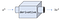Figure 2: A goal is to write a derivative function in Python, taking an expression for a function and returning an expression for its derivative.

Let’s start by thinking differently about algebraic expressions, considering them as structured collections of symbols rather than procedural computations.

## Modeling algebraic expressions

Let’s look closer at the function f(x) = (3x2 + x) sin(x), and see how we can break it down into pieces. Once we’ve gone through this exercise conceptually, we’ll see how to translate our results to Python code.

One first observation is that “f” is an arbitrary name for this function. For instance, the right-hand side of this equation expands the same way regardless of what you call it. Because of this, we’re going to focus only the expression that defines the function, which in this case is (3x2 + x) sin(x). An expression is a collection of mathematical symbols — numbers, letters, operations — combined in certain valid ways. Our first goal is to model these symbols and the ways of composing them in Python.

## Breaking an expression into pieces

We can start to model algebraic expressions by breaking them up into smaller expressions. There’s only one meaningful way to break up the expression (3x2 + x) sin(x) it’s the product of 3x2 + x and sin(x).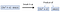Figure 3: A meaningful way to break up an algebraic expression into two smaller expressions.

By contrast, we can’t split this expression at the plus sign. We could make sense of the expressions on either side of the plus sign if we tried, but the result isn’t equivalent to the original expression.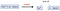Figure 4: It doesn’t make sense to split the expression up around the plus sign. The original expression isn’t the sum of 3x2 and x · sin(x).

If we look at the expression 3x2 + x, it can be broken up into a sum: 3x2 and x. The conventional order of operations tells us that 3x2 is the product of 3 and x2, not 3x raised to the power of 2.

Multiplication and addition are called operations in arithmetic, but in this article we’ll think of them more abstractly. They are means for taking two (or more) algebraic expressions and sticking them together side-by-side to make a new, bigger algebraic expression. Likewise, they’re valid places where we can break up an existing algebraic expression into smaller ones. In the terminology of functional programming, functions combining smaller objects into bigger ones like this are often called combinators. Here are some of the combinators implied in our expression.

• 3x2 is the product of the expressions “3” and “x2”
• x2 is a power — one expression “x” raised to the power of another expression “2”.
• The expression sin(x) is a function application. Given the expression “sin” and the expression “x”, sin(x) is a new expression.

A variable “x”, a number “2”, or a function name “sin” can’t be broken down further. To distinguish them from combinators, these are called elements. The lesson here is that although “(3x2 + x) sin(x)” is only a bunch of symbols printed on this page, the symbols are combined in certain ways to convey mathematical meaning.

## Building an expression tree

The elements “3,” “x,” “2,” and “sin,” along with the combinators of adding, multiplying, raising to a power, and applying a function, are sufficient to rebuild the whole of the expression (3x2 + x) sin(x). Let’s go through the steps and draw the structure we end up building.

One of the first constructions we can put together is x2, which combines x and 2 with the power combinator.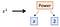Figure 5: Combining x and 2 with the power combinator to represent the bigger expression x2.

A good next step is to combine x2 with the number 3 via the product combinator, to get the expression 3x2: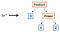Figure 6: Combining the number 3 with a power to model the product 3x2.

This construction is two layers deep: one of the inputs to the product combinator is itself a combinator. As we add more of the terms of the expression, it gets even deeper. The next step is adding the element “x” to 3x2 using the sum combinator, representing the operation of addition.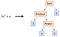Figure 7: Combining the expression 3x2 with the element x with the sum combinator to get 3x2 + x.

Finally, we need to use the function application combinator to apply “sin” to “x” and then the product combinator to combine sin(x) with what we’ve built.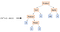Figure 8: A complete picture showing how to build (3x2 + x) · sin(x) from elements and combinators.

You may recognize the structure we’ve built as a tree. The “root” of the tree is the product combinator, with two branches coming out of it. Each combinator appearing further down the tree adds additional branches, until you reach the elements which are “leaves” and have no branches. Any algebraic expression built with numbers, variables, and named functions as elements and operations as combinators correspond to a distinctive tree which reveals its structure. The next thing we can do is build the same tree in Python.

## Translating the expression tree to Python

We can think of each combinator as a function which takes one or more expressions as inputs and returns a new expression as an output. In practice, it is also useful to think of a combinator as a named container that holds its inputs. For instance, the result of applying the power combinator to x and 2 should be some object that holds both x and 2 as data. For a power expression like x2, x is called the base and 2 is called the exponent. A Python class equipped to hold a base and an exponent might look like this:

`class Power():     def __init__(self,base,exponent):         self.base = base         self.exponent = exponent`

We could then write `Power(“x”,2)` in Python to represent the expression x2. Rather than using raw strings and numbers, I’ll create special classes to represent numbers and variables.

`class Number():     def __init__(self,number):         self.number = number   class Variable():     def __init__(self,symbol):         self.symbol = symbol`

This may seem like unnecessary overhead, but it’s useful to be able to distinguish `Variable(“x”)`, which means the letter “x” interpreted as a variable, from the string “x”, which is merely a string. Using these three classes, we can model the expression x2 as

`Power(Variable("x"),Number(2))`

Each of our combinators can be implemented as an appropriately named class which stores the data of whatever expressions it combines. For instance, a product combinator can be a class that stores two expressions which are meant to be multiplied together.

`class Product():     def __init__(self, exp1, exp2):         self.exp1 = exp1         self.exp2 = exp2`

The product 3x2 can be expressed using this combinator as follows.

`Product(Number(3),Power(Variable("x"),Number(2)))`

After introducing the rest of the classes that we need, we can model the whole original expression, as well as a practically infinite list of other possibilities.

`class Sum():     def __init__(self, *exps): #<1>         self.exps = exps`
1. We allow a Sum of any number of terms, meaning we could add two or more expressions together at once.
2. A Function object stores a string which is its name, like “sin”.
3. An Apply combinator stores a function and the argument it’s applied to.
4. I used extra whitespace to make the structure of the expression clearer to see.

This is a faithful representation of the original expression: (3x2+ x) sin(x). By that I mean that we could look at this Python object and see that it describes the algebraic expression, and not a different one. For another expression, like

`Apply(Function("cos"),Sum(Power(Variable("x"),Number("3")), Number(-5)))`

We can read it carefully and see that it represents a different expression: cos(x3 + −5). In the exercises that follow, you can practice translating some Algebraic expressions to Python and vice versa. You’ll see it can be tedious to type out the whole representation of an expression. The good news is that once it’s encoded in Python, the manual work is over. The next thing we’ll see is how to write Python functions to automatically do work with our expressions.

## Exercises

Exercise: Draw the expression ln(yz) as a tree built out of elements and combinators from this section.

Solution: The outermost combinator is an “Apply”. The function being applied is “ln”, the natural logarithm, and the argument is yz. In turn, yz is a power, with base y and exponent z. The result looks like this:

Exercise: Translate the expression from the previous exercise to Python code. Write it both as a Python function and as a data structure built from elements and combinators.

Solution: You can think of ln(yz) as a function of two variables, y and z. It translates directly to Python (where ln is called log):

`from math import log def f(y,z):     return log(y**z)`

The expression tree is built like this:

`Apply(Function("ln"), Power(Variable("y"), Variable("z")))`

Exercise: What’s the expression represented by Product(Number(3),Sum(Variable(“y“),Variable(“z“))) ?

Solution: This represents 3·(y + z). Notice that the parentheses are necessary because of order of operations.

Exercise: Implement a “`Quotient`” combinator representing one expression divided by another. How do you represent the following expression?

Solution: A quotient combinator needs to store two expressions: the top expression is called the numerator and the bottom is called the denominator.

`class Quotient(Expression):     def __init__(self,numerator,denominator):         self.numerator = numerator         self.denominator = denominator`

The sample expression is the quotient of the sum a + b with the number 2:

`Quotient(Sum(Variable("a"),Variable("b")),Number(2))`

Exercise: Implement a “`Difference`” combinator representing one expression subtracted from another. How can you represent the expression b2 – 4ac ?

Solution: The `Difference` combinator needs to store two expressions, and it represents the second subtracted from the first.

`class Difference(Expression):     def __init__(self,exp1,exp2):         self.exp1 = exp1         self.exp2 = exp2`

The expression b2–4ac is the difference of the expressions b2 and 4ac, and it’s represented as follows.

`Difference(     Power(Variable('b'),Number(2)),     Product(Number(4),Product(Variable('a'), Variable('c'))))`

Exercise: Implement a “Negative” combinator, representing the negation of an expression. For example, the negation of x2 + y is −(x2 + y). Represent the latter expression in code using your new combinator.

Solution: The Negative combinator is a class which holds one expression.

`class Negative():     def __init__(self,exp):`

To negate x2 + y we pass it in to the Negative constructor.

`Negative(Sum(Power(Variable("x"),Number(2)),Variable("y")))`

Exercise: Add a Function called “sqrt” representing a square root, and use it to encode the following formula:

Solution: To save some typing, we can name our variables and square root function up front.

`A = Variable('a') B = Variable('b') C = Variable('c') Sqrt = Function('sqrt')`

Then it’s a matter of translating the algebraic expression into the appropriate structure of elements and combinators. At the highest level, you can see this is a quotient of a sum (on top) and a product (on the bottom).

`Quotient(     Sum(         Negative(B),         Apply(             Sqrt,             Difference(                 Power(B,Number(2)),                 Product(Number(4), Product(A,C))))),     Product(Number(2), A))`

Mini-project: Create an abstract base class called Expression and make all of the elements and combinators inherit from it. For instance, `class Variable()` should become `class Variable(Expression)`. Then, overload the Python arithmetic operations +, -, *, and / to produce Expression objects. For instance, the code 2 * Variable(“x”) + 3 should yield: `Sum(Product(Number(2), Variable(“x”)), Number(3))`.

Solution: See source code.

Putting a symbolic expression to work

For the function we’ve been studying this far, we wrote down a Python function that computes it:

`def f(x):     return (3*x**2 + x)*sin(x)`

As an entity in Python, this function is only good for one thing: returning an output value for a given input value x. The value “f” in Python doesn’t make it particularly easy to programmatically answer the questions we asked at the beginning of the article, like whether “f” depends on its input, whether “f” contains a trigonometric function, or what the body of “f” would look like if it were expanded algebraically. In this section we’ll see that once we’ve translated the expression into a data structure in Python, built from elements and combinators, we can answer all of these questions and more.

## Finding all the variables in an expression

It’s clear that the output value of f(x) depends on the input value

, and by comparison g(x) = 7 doesn’t. Some cases are tricky like h(x) = x − x, p(x) = 4x2 — (2x + 1)(2x − 1), or q(x) = sin(x)2 + cos(x)2 which also don’t depend on the value of x (can you see why?) but we won’t focus on these. Let’s ask a more general question: given an expression, which distinct variables appear in it? We can write a Python function `distinct_variables` which takes an expression (meaning any of our elements or combinators) and returns a Python set containing the variables.

If our expression is an element, like z or 7, the answer is clear. An expression which is only a variable contains one distinct variable, but an expression which is only a number contains no variables at all. We’d expect our function to behave accordingly:

`>>> distinct_variables(Variable("z")) {'z'}`

The situation is more complicated when the expression is built up from some combinators, like y · z + x2. It’s easy for a human to read off all the variables, which are y, z, and x, but how do we extract these from the expression in Python? This is a Sum combinator, representing the sum of y · z and xz. The first expression in the sum has y and z, but the second has x and z. The sum contains all of the variables in these two expressions.

This suggests that we should use a recursive solution: the `distinct_variables` for a combinator are the collected `distinct_variables` for each of the expressions it contains. The end of the line are the variables and numbers, which obviously contain either one or zero variables. To implement the `distinct_variables` function, we need to handle the case of every element and combinator which is a valid expression.

`def distinct_variables(exp):     if isinstance(exp, Variable):         return set(exp.symbol)     elif isinstance(exp, Number):         return set()     elif isinstance(exp, Sum):         return set().union(*[distinct_variables(exp) for exp in exp.exps])     elif isinstance(exp, Product):         return distinct_variables(exp.exp1).union(distinct_variables(exp.exp2))     elif isinstance(exp, Power):         return distinct_variables(exp.base).union(distinct_variables(exp.exponent))     elif isinstance(exp, Apply):         return distinct_variables(exp.argument)     else:         raise TypeError("Not a valid expression.")`

As expected, our f_expression contains only the variable x:

`>>> distinct_variables(f_expression) {'x'}`

If you’re familiar with the tree data structure, you’ll recognize this as a recursive traversal of the expression tree. By the time this function has completed running, it has called `distinct_variables` on every expression contained in the target expression, which are all of the nodes in the tree. That ensures that we’ve seen every variable, and we get the correct answers we expect. In the exercises at the end of the section, you can use a similar approach to find all of the numbers or all of the functions.

## Evaluating an expression

Now we’ve got two representations of the same mathematical function f(x). One is the Python function, f, which is good for evaluating the function at a given input value of x. The new one is this tree data structure that describes the structure of the expression defining f(x). It turns out the latter representation has the best of both worlds — we can use it to evaluate f(x) as well, with only a little more work.

Mechanically, evaluating a function f(x) at, say, x = 5 means plugging in the value of 5 for x everywhere and then doing the arithmetic to find the result. If the expression were f(x) = x, plugging-in x = 5 tells us f(5) = 5. Another simple example is g(x) = 7, where plugging-in 5 in place of x has no effect — there are no appearances of x on the right-hand side, and the result of g(5) is 7.

The code to evaluate an expression in Python is similar to the code we wrote to find all variables. Instead of looking at the set of variables which appear in each sub-expression, we need to evaluate each sub-expression, then the combinators tell us how to combine these results to get the value of the whole expression.

The starting data we need are the values that need to be plugged-in and which variables they’ll replace. For instance an expression in two different variables like z(x,y) = 2xy3 need two values to get a result, for instance x = 3 and y = 2. In computer science terminology, these are called variable bindings. With these, we can evaluate the sub-expression y3 as (2)3 which equals 8. Another sub-expression is 2x, which evaluates to 2 · (3) = 6. These two are combined with the product combinator, and the value of the whole expression is the product of 6 and 8, or 48.

As we translate this procedure into Python code, I’m going to show you a slightly different style than in the previous example. Rather than having a separate “evaluate” function, we can add an evaluate method to each class representing an expression. To enforce this, we can create an abstract Expression base class with an abstract evaluate method, and have each kind of expression inherit from it. Here’s an Expression base class, complete with an evaluate method.

`from abc import ABC, abstractmethod   class Expression(ABC):     @abstractmethod     def evaluate(self, **bindings):         pass`

Because an expression may contain more than one variable, I set it up to allow you to pass in the variable bindings as keyword arguments. For instance, the bindings {x : 3, y : 2} mean “substitute 3 for x and 2 for y.” This gives us some nice syntactic sugar when evaluating an expression. If z represents the expression 2xy3 then once we’re done, we’ll be able to execute the following.

`>>> z.evaluate(x=3,y=2) 48`

This far, we’ve only got an abstract class. We need to do the work of having all of our expression classes inherit from Expression. For instance, a Number instance is an expression, as a number on its own, like “7,” is a valid expression. Regardless of the variable bindings provided, a number evaluates to itself.

`class Number(Expression):     def __init__(self,number):         self.number = number     def evaluate(self, **bindings):         return self.number`

For instance, evaluating Number(7).evaluate(x=3,y=6,q=-15) or any other evaluation for that matter, returns the underlying number: seven.

Handling variables is also simple. If we’re looking at the expression Variable(“x”), we only need to consult the bindings and see what number the variable “x” is set to. When we’re done, we should be able to run Variable(“x”).evaluate(x=5) and get 5 as a result. If we can’t find a binding for “x” then we can’t complete the evaluation and we need to raise an exception. Here’s the updated definition of the Variable class:

`class Variable(Expression):     def __init__(self,symbol):         self.symbol = symbol     def evaluate(self, **bindings):         try:             return bindings[self.symbol]         except:             raise KeyError("Variable '{}' is not bound.".format(self.symbol))`

With the elements handled, we need to turn our attention to the combinators. (Note that we won’t consider a Function object an Expression on its own, because a function like “sin” isn’t a standalone expression. It can only be evaluated when it’s given an argument, in the context of an Apply combinator.) For a combinator like Product, the rule used to evaluate it is simple: evaluate both expressions contained in the product, and then multiply the results together. No substitution needs to be performed in the product, but we’ll pass the bindings along to both sub-expressions in case either contains a Variable.

`class Product(Expression):     def __init__(self, exp1, exp2):         self.exp1 = exp1         self.exp2 = exp2     def evaluate(self, **bindings):         return self.exp1.evaluate(**bindings) * self.exp2.evaluate(**bindings)`

With these three classes updated with evaluate methods, we can now evaluate any expression built out of variables, numbers, and products. For instance:

`>>> Product(Variable("x"), Variable("y")).evaluate(x=2,y=5) 10`

Similarly, we can add an “evaluate” method to the Sum, Power, Difference, or Quotient combinators. Once we evaluate their sub-expressions, the name of the combinator tells us which operation we use to get the overall result. The “Apply” combinator works a bit differently, and it deserves some special attention. We need to dynamically look at a function name like “sin” or “sqrt” and figure out a recipe to compute its value. This can be done in a few ways, but I chose keeping a dictionary of known functions as data on the Apply class. As a first pass, we can make our evaluator aware of three named functions:

`_function_bindings = {     "sin": math.sin,     "cos": math.cos,     "ln": math.log }   class Apply(Expression):     def __init__(self,function,argument):         self.function = function         self.argument = argument     def evaluate(self, **bindings):         return _function_bindings[self.function.name](self.argument.evaluate(**bindings))`

You can practice writing the rest of the “evaluate” methods yourself, or find them in the source code. Once you’ve got all of them fully implemented, you’ll be able to evaluate our f_expression.

`>>>  f_expression.evaluate(x=5) -76.71394197305108`

The result here isn’t important, only the fact that it’s the same as what the ordinary Python function f(x) gives us.

`>>> f(x) -76.71394197305108`

Equipped with the evaluate function, our Expression objects can do the same work as their corresponding ordinary Python functions.

## Expanding an expression

Many other things can be done with our expression data structures. In the exercises, you can try your hand building a few more Python functions which manipulate expressions in different ways. I’ll show you one more example for now, which I mentioned at the beginning of the article: expanding an expression. What I mean by this is taking any product or power of sums and carrying it out.

The relevant rule of algebra is the distributive property of sums and products. This rule says that a product of the form (a + b)c is equal to ac + bc and similarly that x(y + z) = xy + xz. For instance, our expression (3x2 + x)sin(x) is equal to 3x2sin(x) + xsin(x), which is called the expanded form of the first product. You can use this rule several times to expand more complicated expressions, for instance:

As you can see, expanding a short expression like (x + y)3 can be a lot of writing. In addition to expanding this expression, I also simplified the result a bit, rewriting some products that look like xyx or xxy as x2y, for instance. Then I further simplified by combining like terms, noting that there were three summed copies each of x2y and y2x, and grouping them together into 3x2y and 3y2x. In this example, I’ll only look at how to do the expanding — you can implement simplification as an exercise.

We can start by adding an abstract `expand` method to the Expression base class.

`class Expression(ABC):     ...     @abstractmethod     def expand(self):         pass`

If an expression is a variable or number, it’s already expanded. For these cases, the expand method returns the object itself. For instance:

`class Number(Expression):     ...     def expand(self):         return self`

Sums are already considered to be expanded expressions, but the individual terms of a sum may not be expanded. For instance 5 + a(x + y) is a sum in which the first term, 5, is fully expanded but the second term, a(x + y) isn’t. To expand a sum, we need to expand each of the terms and sum them.

`class Sum(Expression):     ...     def expand(self):         return Sum(*[exp.expand() for exp in self.exps])`

The same procedure works for function application. We can’t expand the Apply itself, but we can expand the arguments to the function. This expands an expression like sin(x(y + z)) to sin(xy + xz).

`class Apply(Expression):     ...     def expand(self):         return Apply(self.function, self.argument.expand())`

The real work comes when we’re expanding products or powers, where the structure of the expression changes completely. As an example, a(b + c) is a product of a variable with a sum of two variables, and its expanded form is ab + ac, the sum of two products of two variables each. To implement the distributive law, we have to handle three cases: the first term of the product may be a sum, the second term may be a sum, or neither of them may be sums. In the latter case, no expanding is necessary.

`class Product(Expression):     ...     def expand(self):         expanded1 = self.exp1.expand() #<1>         expanded2 = self.exp2.expand()         if isinstance(expanded1, Sum): #<2>             return Sum(*[Product(e,expanded2).expand() for e in expanded1.exps])         elif isinstance(expanded2, Sum): #<3>             return Sum(*[Product(expanded1,e) for e in expanded2.exps])         else:             return Product(expanded1,expanded2) #<4>`
1. First, expand both terms of the product
2. If the first term of the product is a `Sum`, take the product with each of its terms multiplied by the second term of the product. Call expand on the result in case the second term of the product is also a `Sum`.
3. If the second term of the product is a `Sum`, multiply each of its terms by the first term of the product.
4. Otherwise, neither term is a `Sum`, and the distributive law doesn’t need to be invoked.

With all of these methods implemented, we can test the expand function. With the appropriate implementation of `__repr__` (see the exercises) you can see it in action in an interactive session. It correctly expands (a + b)(x + y) to ax + ay + bx + by :

`>>> Product(Sum(A,B),Sum(Y,Z)).expand() Product(Sum(Variable("a"),Variable("b")),Sum(Variable("x"),Variable("y")))`

And our expression (3x2 + x)sin(x) expands correctly to 3x2sin(x) + xsin(x):

`>>> f_expression Sum(Product(Product(3,Power(Variable("x"),2)),Apply(Function("sin"),Variable("x"))),Product(Variable("x"),Apply(Function("sin"),Variable("x"))))`

At this point we’ve written some Python functions which do algebra for us, not only arithmetic. This kind of modeling of expressions is called symbolic programming or more specifically computer algebra. There are a lot of fun applications, and you can learn more about them in the book.